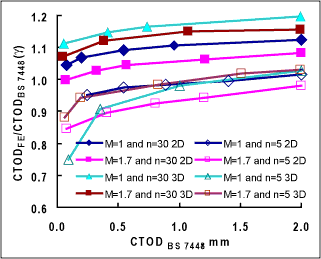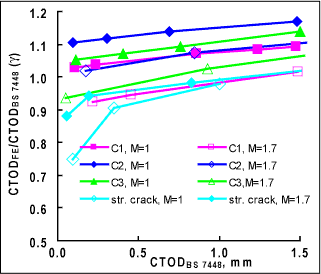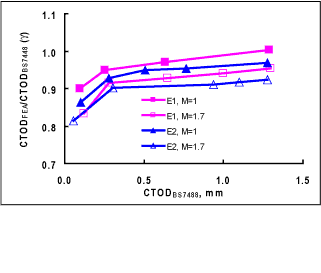Subscribe to our newsletter to receive the latest news and events from TWI:

# Effects of Geometry and Tensile Properties on J and CTOD

## FEA Investigations into the Effects of Geometry and Tensile Properties on J and CTOD in Standard Fracture Specimens

Liwu Wei and Henryk G Pisarski

Paper presented at ESIA9 - 9th International Conference on Engineering Structural Integrity Assessment, 15 - 19 October 2007, Beijing, China.

Current standard CTOD/J testing procedures ( e.g. BS 7448) specify the use of deeply notched specimens loaded in tension or bending. The load versus displacement curve obtained from the specimen is used to estimate J or CTOD. The validity of the equations given in the standards is questioned when they are used for welds, where there is strength inhomogeneity, and there are variations in specimen geometry. Using 2D and 3D FEA, this work assessed the effects due to crack straightness, specimen geometry and weld to base metal strength mismatch on J & CTOD and how these differ from standard formulations.

## Background

Current standard CTOD/J testing procedures such as BS 7448  specify the use of deeply notched specimens loaded in tension or bending. The load versus displacement curve obtained from the specimen is used to estimate fracture toughness in terms of J or CTOD. The validity of the equations given in the standards is conditional on three assumptions: i) the material is homogeneous, ii) the material behaves in a perfectly plastic manner at high loads and iii) the specimen has a straight crack front with a crack depth to specimen width ratio ranging from a/W = 0.45 to 0.7. However, these assumptions may be violated and the validity of the equations questioned when they are used for welds, where there is strength inhomogeneity and there are variations in specimen geometry. In order to assess the effects due to crack front straightness, specimen geometry and weld to base metal strength mismatch on J & CTOD and how these differ from standard formulations, two and three-dimensional finite element modeling of a standard single edge notch bend specimen has been undertaken.

## Specimens and material properties

A standard (to BS 7488) rectangular section, Bx2B, single edge notch bend (SENB) specimen (B = 25mm) was modeled. The a/W was nominally 0.5. Three different categories of specimen were considered: a specimen with straight crackfront, one with a curved crack front, and curved specimen (along the width, W) with a straight crack front.

Two strain hardening exponents, n=5 and 30 (corresponding to strong work hardening and approximately elastic-perfectly plastic behaviour), were considered and the same strain hardening was applied to the weld and base metal. The weld overmatched the base metal yield strength by 70%, ie the strength mismatch ratio (M) of the yield strength of the weld to that of the base metal was 1.7. The yield strength of the weld and base metals are 996MPa and 586MPa, respectively. The modulus of elasticity and Poisson's ratio were 207GPa and 0.3 for both the weld and base metals. The weld metal and base metal stress-strain curves were modeled using a linear power law stress-strain relationship.

## Codified ctod/j evaluation procedures

The standard procedure for deriving J and CTOD from an experimental record given in BS 7448 is to partition J and CTOD into small-scale yielding and fully plastic components. The small-scale yielding components of J and CTOD are expressed as a function of the stress intensity factor, K. The fully plastic components of J and CTOD are determined, respectively, from the plastic component of the area under the load versus load-line displacement record (U P ), and the plastic component of crack mouth opening displacement (V P ). The details of the equations for the calculation of J and CTOD are given in BS 7448 Parts 1, 2 and 4. In this work, U P and V P used for J and CTOD calculations were evaluated from the corresponding load and displacement curves determined from FEA.

## Modelling

The FE models were constructed in ABAQUS/CAE in conjunction with an in-house developed toolkit for generating different categories of SENB specimens. The FE models used 20-node isoparametric elements for 3D cases and 8-nodeisoparametric elements for 2D cases. For the 2D model, plane strain conditions were assumed. Five rings of cylinder were created to surround the crack front, and the small element size adjacent to the crack front was three thousandths of the crack length so that the accuracy in J and CTOD calculations was ensured.

The J value was directly obtained from the elastic-plastic analysis of the FE models at the mid-plane of the specimen which approximated a plane strain state. Crack mouth opening displacements (CMOD) were extracted from the model output to derive CTOD values. CTOD value was determined by extrapolation of the linear part of the CMOD profile to the crack tip.

## Results and discussion

The general findings of this work have shown larger differences between FEA and BS 7488 estimates of CTOD compared with J estimates. For all the cases investigated, the ratio of J from FEA (J FE ) to J from BS 7448 (J BS 7488 ) varies from 0.91 to 1.16, while CTOD FE /CTOD BS 7488 (termed as γ) varies from 0.70 to 1.26. It was also found that the three different categories of specimen (straight crack, curved crack front and curved specimen)showed a general trend that the ratio γ increased with an increasing CTOD.

In Figure 1, the ratio γis plotted versus CTOD BS 7448 for the specimens with a straight crack front analyzed using 2D and 3D FE models. Results were obtained for strong hardening behaviour (n=5) and approximately perfectly plastic condition (n=30), and strength mismatch of M=1 and M=1.7. Figure 1 shows that for the same work hardening and strength mismatch, the 3D models generally produce higher CTOD values than the 2D models. This was probably a result of lower constraint generated at the mid-plane of a 3Dmodel compared to the fully plane strain conditions assumed in the 2D model. The variations in γ mean that at small CTOD values (~ 0.1mm), the BS7488 equation results in a 25% overestimate of CTOD, whilst at large CTOD values (~ 2mm), CTOD is underestimated by 20%. Strain hardening (n=5 and 30) had a significantly great effect on CTOD than strength mismatch (M=1 and 1.7). High strain hardening (n=5) generally lead to overestimates of CTOD being made when the BS 7488equation was used. Similar conclusions were drawn by Wang et al (1997) using 2D plane strain FE models to analyze SENB specimens. Since erroneous estimates of CTOD are likely to be made when high strain hardening material is tested,caution needs to be applied when using CTOD values for structural integrity assessment. It has been suggested that the error can be reduced if CTOD is derived from CMOD via J. [Wang, 1997] Indeed, such a procedure has been adopted in ASTM E1290 and E 1280. The effect of strength mismatch (M=1.7) on CTOD was to slightly reduce the γ ratio (less than 8% for n=30), which is within the error estimate of ±10% quoted in BS 7488.Fig.1. Plots of CTOD FE /CTOD BS 7488 versus CTOD BS 7488 for straight cracks

Figure 2 shows the CTOD values obtained from specimens with curved crack fronts C1, C2 and C3, together with the results from a straight crack front for comparison. Specimens, C1, C2 and C3, have curved crack fronts deviating from a straight crack by 3.4, 7.7 and 16%, respectively. For these specimens, J and CTOD estimated according to BS 7488 used the weighted average crack length in accordance with the procedure in BS 7488. In comparison with a straight crack front, crack front curvature generally resulted in an increase of γ. The effect of crack front curvature on CTOD was found to depend on M (for n=5). When M=1, γ for the curved cracks exceeded 1 which means that the BS 7448 estimate of CTOD is conservative. However, when M=1.7, γ was < 1 for CTOD < 0.8mm so that the BS 7488 estimates of CTOD are non-conservative. For straight crack fronts, the analyses show that the BS 7488estimates of CTOD are non-conservative and the error is larger for evenly mismatched strength condition (M=1).Fig.2. Plots of CTOD FE /CTOD BS 7488 versus CTOD BS 7488 for curved cracks for n=5

The effect of specimen curvature (along the W dimension) was investigated using specimens E1 and E2 with curvatures of 5%W and 10%W, respectively. As shown in Figure 3, the CTOD values calculated from BS 7448 were higher than those from FEA, with a maximum difference of about 20% at a small CTOD values and a typical difference of between 3% and 10%. This means BS 7448 estimates ofCTOD are non-conservative when specimen curvature is more than 5%W. The maximum allowed by BS 7488 Part 2 is 2.5%W.Fig.3. Plots of CTOD FE /CTOD BS 7488 versus CTOD BS 7488 for curved specimens for n=5

## Conclusions

1. Larger differences between FEA and BS 7448 estimates of CTOD were obtained than for J. For all the cases investigated J FE /J BS 7488 varied from 0.91 to 1.16, while CTOD FE /CTOD BS 7488 varied from 0.70 to 1.26.
2. CTOD was influenced more significantly by strain hardening (n=30 and 5) than by strength mismatch (M=1 and 1.7). Increases in strain hardening and mismatch both contribute to a reduction in the ratio of CTOD FE to CTOD BS 7448 ( γ). This implies that CTOD calculated according to BS 7488 will be overestimated under the conditions of high strain hardening and mismatch.
3. Curved crack fronts were found to increase the ratio γ. Specimen curvature in the width dimension (W) caused a decrease in the ratio γ.

## Acknowledgements

This work was funded by a TWI exploratory research programme. Thanks are also due to Dr Simon Smith of TWI for his helpful discussions.

## References

1. British Standards Institute, Fracture mechanics toughness tests: Part 2. Method for determination of K IC , critical CTOD and critical J values of welds in metallic materials, BS 7448: Part 2: 1997.
2. Wang, Y.-Y., Reemsynder H.S. & Kirk, M.T., 'Inference of equations for fracture toughness testing: numerical analysis and experimental verification', ASTM STP 1321, 1997.